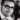# Javascript Basic Reference

February 07, 2021

## Declaring Variables

`const myVar = 1` can’t reassign, block scoped

`let myVar = 2` can reassign, block scoped

`var myVar = 3` can reassign, global unless in function

## Math Operators

• `+, -` Add and Substract
• `*, /` multiply and divide
• `**` exponent
• `++` increment (add one)
• `--` decrement (subtract one)
• `+=` add and reassign
• `-=` subtract and reassign
• `*=` multiply and reassign
• `/=` divide and reassign
• `%` modulo/modulus
• `Math.random()` generate random number (0-1)
• `Math.ceil()` round a number up
• `Math.floor()` round a number down
• `Math.round()` round a number
• `Math.sqrt()` square root of number
• `Math.PI` The numerical value of PI

## Boolean Operators

• `< >` greater than, less than
• `<= >=` less equal, greater equal
• `== ===` equality, strict equality
• `!= !==` inequality, strict inequality
• `&&` and
• `||` or

## Conditionals

• IF statement
``````if (expression){
//code if true
} else if (expression){
//code if second expression is true
} else {
//code if all expressions are false
}``````
• Ternary Operator
``expression ? resultIfTrue : resultIfFalse``
• Switch Statements
``````switch(value){
case possibleMatch:
//code if match
break

default:
//code if no case matches
break
}``````

## Loops

• while loops
``````while(expression){
//code to repeat as long as expression is true
}``````
• for loops
``````for(initiateCounter; expression; adjustCounter){
//code will that will as long as expression is true
}``````
• for of loops
``````for (item of iterable){
//code that will run for each item in iterable (array, set, map) and on each loop the current item will be stored in "item"
}``````
• for in loops (for objects)
``````for(key in object){
//loops over object keys, access value with object[key]
}``````
• forEach Array Method
``````Array.forEach((item) => {
// code to run for each item in the array, each item will take turns being stored in "item"
})``````

## Functions

``````function myFunc(){
//code to run when function invoked
}``````

hoisted, can use this keyword

``````const myFunc = function(){
//code to run when function is invoked
}``````

not hoisted, can use this keyword

``````const myFunc = () => {
//code to run when the function is invoked
}``````

not hoisted, can’t use this keyword

## Arrays

• `const arr = [1,2,3]` declare an array
• `arr` access an array value
• `const [one, two, three] = arr` array destructuring
• `const arrCopy = [...originalArr]` copy array
• `const dupeFree = [...new Set(arr)]` remove duplicates

## Objects

• `const obj = {one: 1, two: 2}` declare an object
• `obj.one` accessing property with dot notation
• `obj["two"]` accessing property with square brackets
• `const {one, two} = obj` object destructuring
• `const objCopy = {...originalObj}` copy an object

## DOM Manipulation

• `document.querySelector('h1')` return the first matching node
• `document.querySelectorAll('h1')` return array of all matching nodes
• `node.innerHTML` property containing string to parsed as html in the node
• `node.innerText` property containing string to be parsed as text in the node
• `node.style` object containing all CSS properties of ndoe
• `parentNode.appendChild(childNode)` append a node as a child of a node
• `node.addEventListener("click", someFunction)` add event listener, function will run whenever specified event occurs
• `document.createElement("h1")` create a nodeWritten by Alex Merced Developer from devNursery.com and alexmercedcoder.dev You should follow him on Twitter and checkout his articles on LogRocket.